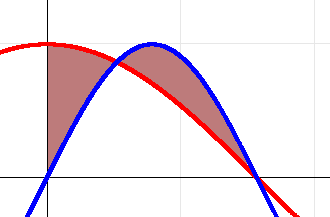# Area between curves

Gold Member

## Homework Statement

Find the area of the region bounded by:
y=cosx, y=sin2x, 0, x=pi/2

## The Attempt at a Solution

I made a graph. I believe I'm trying to find the area I shaded.red=cos(x), blue=sin(2x)

I need to find the intersection point so I will know the limits of my 2 integrals.

cosx = sin2x

But I don't know how to do this. There should be an infinate number of intersections, but I am only interested in the one that appears to happen around x=1/2 and the next one at what appears to be pi/2.

I can verify with my calculator that cos(pi/2) and sin(2pi/2) both equal 0, and that the right limit given by the problem is indeed the intersection, but that is not the case for the 1st intersection. How do I solve this? And what if the book gave the right limit as x=2. My method of eyeballing it and verifying my guess with the calculator would fail.

Assuming I find the intersection point, the next thing I was going to do is:
$$\left( {\int_0^{???} {\cos x} \,dx\, - \,\int_0^{???} {\sin 2x} \,dx} \right)\, + \,\,\left( {\int_{???}^{\pi /2} {\sin 2x\,dx} - \int_{???}^{\pi /2} {\cos x\,dx} } \right)$$

## The Attempt at a Solution

Gib Z
Homework Helper
sin(2x)=2 sinx cos x
cos x= 2sinx cos x
1=2 sin x
sin x =1/2
So the solution is arcsin (1/2). What value of sin x gives us 1/2? pi/6 of course.

Thats the systematic way of doing it, you may also have done cos x= sin (pi/2 -x) so equate them, sin (pi/2 - x)=sin 2x, 2x=pi/2 - x, 3x=pi/2, x=pi/6.

The 2nd way is only useful for angles less than pi/2, which means it can be used here.

Now that you have both intersection points just integrate as how you were going to do next, knowing the ???'s is pi/6.

Gold Member

sin(2x)=2 sinx cosx
I guess that's just a trig identity I forgot? Playing with it on my calculator, I can see that it works for all values of x.

cosx = 2 sinx cosx
Playing with this on my calculator, it does not work for all values of x. Is this a trig identity, or specific to this problem?

If specific to this problem, from sin(2x)=2 sinx cosx i get
cosx = sin(2x)/2sin x

How did you get cosx = 2 sinx cosx ?

Gib Z
Homework Helper
Sin 2x = 2sinx cos x is a identity you just forgot.

Cos x= 2sinx cos x is specific to the problem. When we want to find points of intersection, we set the 2 functions to be equal, then solve. The functions we have are sin 2x and cos x. Since sin 2x=2sinx cos x for all x, we sub that in, then we have to solve cos x=2sinx cos x.

I dont know why you got cos x= sin(2x)/2 sin x, thats not needed.

cos x = 2sin x cos x

Divide both sides by 2 cos x, sin x=1/2. Solving for that, arcsin 1/2=pi/6

Gib Z
Homework Helper
The Indentity can be easily seen from expanding the formula for sin(x+y), letting y=x.